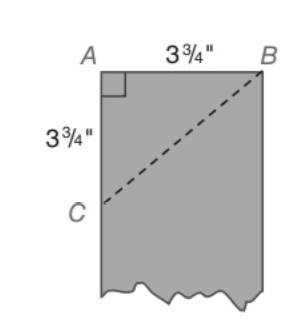Chapter 5.5, Problem 23E### Elementary Geometry for College St...

6th Edition
Daniel C. Alexander + 1 other
ISBN: 9781285195698

#### Solutions

Chapter
Section### Elementary Geometry for College St...

6th Edition
Daniel C. Alexander + 1 other
ISBN: 9781285195698
Textbook Problem
16 views

# A carpenter is working with a board that is 3 3 4 in. wide. After marking off a point down the side of length 3 3 4 in., the carpenter makes a cut along B C ¯ with a saw. What is the measure of the angle ( ∠ A C B ) that is formed?To determine

To find:

The measure o the angle ACB that is formed when a carpenter working with a board that is 334 in. wide. After marking off a point down the is a of length 334 in the carpenter makes a ct along BC with a saw.

Explanation

Approach:

For a right triangle for which the measure of the interior angles 45°, 45°, and 90°, if ‘a’ is the length of measure of one of the leg; opposite to the angle 45°, then the length of the other two sides is given by

Length of the other leg =a

Length of the hypotenuse =a2

In general

Length of the legs are equal.

Length of the hypotenuse =2× (Length of one of the legs)

Calculation:

Given,

A triangular piece of board that cut off from a board of wide 334 in along its diagonal that meets the opposite side of the board 334 in down the side of length

### Still sussing out bartleby?

Check out a sample textbook solution.

See a sample solution

#### The Solution to Your Study Problems

Bartleby provides explanations to thousands of textbook problems written by our experts, many with advanced degrees!

Get Started

#### Convert the expressions in Exercises 6584 to power form. xy23

Finite Mathematics and Applied Calculus (MindTap Course List)

#### Demand The demand function for a product is given by where x is the number o...

Mathematical Applications for the Management, Life, and Social Sciences

#### Find the remaining trigonometric ratios. 30. tan = 2, 02

Single Variable Calculus: Early Transcendentals, Volume I

#### True or False: is a convergent series.

Study Guide for Stewart's Multivariable Calculus, 8th